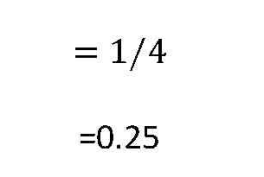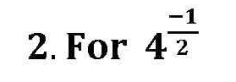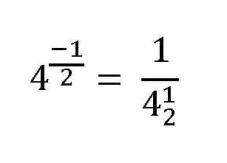Negative Exponents

It determines how many times a number is to be divided.

Formula for Negative Exponents

The general formula for negative exponents iswhere n is the positive exponent and -n is the negative exponent with base a .

Description

Negative exponents are easy way to convert division problems into multiplication problems as numbers can be presented in powers of decimal or fraction without need to write them in decimal or fraction forms respectively. The above formula says a negative exponent can be converted into positive by changing its position in fractional line i.e. moving the exponents present in numerator to denominator or vice versa.

Rules for negative exponents

The below rules are applicable for all negative fractional exponents or non-fractional exponents

1.Convert the negative exponent number into positive exponent number by taking its reciprocal

2.Solve the positive exponent number

3.Subtract the exponents with similar base in both numerator and denominator and add the exponents with similar base in both numerator and denominator

Simplifying Negative Exponents

Let’s use the above rules to solve the following examplesSolution

a. Taking reciprocal of the given expression [Rule 1] , we haveb. Simplifying the positive exponent [Rule 2] , we haveSolution

a. Taking reciprocal of the given expression [Rule 1] , we haveb.Simplifying the positive exponent [Rule 2] , we haveDividing negative exponents

Exponents having same base are subtracted in division and added in multiplicationSolution

a. Taking reciprocal of the given expression [Rule 1] , we haveb. Implementing Rule 3 , we got